x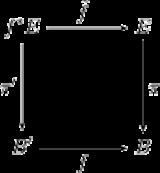Pullback bundleEncyclopedia
In mathematics
Mathematics
Mathematics is the study of quantity, space, structure, and change. Mathematicians seek out patterns and formulate new conjectures. Mathematicians resolve the truth or falsity of conjectures by mathematical proofs, which are arguments sufficient to convince other mathematicians of their validity...

, a pullback bundle or induced bundle is a useful construction in the theory of fiber bundle
Fiber bundle
In mathematics, and particularly topology, a fiber bundle is intuitively a space which locally "looks" like a certain product space, but globally may have a different topological structure...

s. Given a fiber bundle π : E → B and a continuous map f : B′ → B one can define a "pullback" of E by f as a bundle f*E over B′. The fiber of f*E over a point x in B′ is just the fiber of E over f(x). Thus f*E is the disjoint union
Disjoint union
In mathematics, the term disjoint union may refer to one of two different concepts:* In set theory, a disjoint union is a modified union operation that indexes the elements according to which set they originated in; disjoint sets have no element in common.* In probability theory , a disjoint union...

of all these fibers equipped with a suitable topology
Topological space
Topological spaces are mathematical structures that allow the formal definition of concepts such as convergence, connectedness, and continuity. They appear in virtually every branch of modern mathematics and are a central unifying notion...

.

## Formal definition

Let π : E → B be a fiber bundle with abstract fiber F and let f : B′ → B be a continuous map. Define the pullback bundle by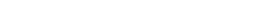and equip it with the subspace topology
Subspace topology
In topology and related areas of mathematics, a subspace of a topological space X is a subset S of X which is equipped with a natural topology induced from that of X called the subspace topology .- Definition :Given a topological space and a subset S of X, the...

and the projection map π′ : f*E → B′ given by the projection onto the first factor, i.e.,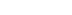The projection onto the second factor gives a map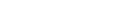such that the following diagram commutes
Commutative diagram
In mathematics, and especially in category theory, a commutative diagram is a diagram of objects and morphisms such that all directed paths in the diagram with the same start and endpoints lead to the same result by composition...

:If (U, φ) is a local trivialization of E then (f−1U, ψ) is a local trivialization of f*E where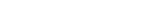It then follows that f*E is a fiber bundle over B′ with fiber F. The bundle f*E is called the pullback of E by f or the bundle induced by f. The map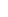is then a bundle morphism covering f.

## Properties

Any section
Section (fiber bundle)
In the mathematical field of topology, a section of a fiber bundle π is a continuous right inverse of the function π...

s of E over B induces a section of f*E, called the pullback section f*s, simply by defining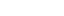.

If the bundle E → B has structure group G with transition functions tij (with respect to a family of local trivializations {(Ui, φi)} ) then the pullback bundle f*E also has structure group G. The transition functions in f*E are given by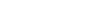If E → B is a vector bundle
Vector bundle
In mathematics, a vector bundle is a topological construction that makes precise the idea of a family of vector spaces parameterized by another space X : to every point x of the space X we associate a vector space V in such a way that these vector spaces fit together...

or principal bundle
Principal bundle
In mathematics, a principal bundle is a mathematical object which formalizes some of the essential features of the Cartesian product X × G of a space X with a group G...

then so is the pullback f*E. In the case of a principal bundle the right action
Group action
In algebra and geometry, a group action is a way of describing symmetries of objects using groups. The essential elements of the object are described by a set, and the symmetries of the object are described by the symmetry group of this set, which consists of bijective transformations of the set...

of G on f*E is given by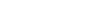It then follows that the mapis equivariant
Equivariant
In mathematics, an equivariant map is a function between two sets that commutes with the action of a group. Specifically, let G be a group and let X and Y be two associated G-sets. A function f : X → Y is said to be equivariant iffor all g ∈ G and all x in X...

and so defines a morphism of principal bundles.

In the language of category theory
Category theory
Category theory is an area of study in mathematics that examines in an abstract way the properties of particular mathematical concepts, by formalising them as collections of objects and arrows , where these collections satisfy certain basic conditions...

, the pullback bundle construction is an example of the more general categorical pullback. As such it satisfies the corresponding universal property
Universal property
In various branches of mathematics, a useful construction is often viewed as the “most efficient solution” to a certain problem. The definition of a universal property uses the language of category theory to make this notion precise and to study it abstractly.This article gives a general treatment...

.

The construction of the pullback bundle can be carried out in subcategories of the category of topological spaces, such as the category of smooth manifolds. The latter construction is useful in differential geometry and topology
Differential geometry and topology
Differential geometry is a mathematical discipline that uses the techniques of differential and integral calculus, as well as linear and multilinear algebra, to study problems in geometry. The theory of plane and space curves and of surfaces in the three-dimensional Euclidean space formed the basis...

Examples: It is illuminating to consider the pullback of the degree 2 map from the circle to itself over the degree 3 or 4 map from the circle to itself. In such examples one sometimes gets a connected and sometimes disconnected space, but always several copies of the circle.

## Bundles and sheaves

Bundles may also be described by their sheaves of sections
Sheaf (mathematics)
In mathematics, a sheaf is a tool for systematically tracking locally defined data attached to the open sets of a topological space. The data can be restricted to smaller open sets, and the data assigned to an open set is equivalent to all collections of compatible data assigned to collections of...

. The pullback of bundles then corresponds to the inverse image of sheaves
Inverse image functor
In mathematics, the inverse image functor is a contravariant construction of sheaves. The direct image functor is the primary operation on sheaves, with the simplest definition. The inverse image exhibits some relatively subtle features.-Definition:...

, which is a contravariant functor. A sheaf, however, is more naturally a covariant object, since it has a pushforward
Pushforward
The notion of pushforward in mathematics is "dual" to the notion of pullback, and can mean a number of different, but closely related things.*Pushforward : the differential of a smooth map between manifolds, and the "pushforward" operations it defines.**Pushforward #Pushforward of vector...

, called the direct image of a sheaf. The tension and interplay between bundles and sheaves, or inverse and direct image, can be advantageous in many areas of geometry. However, the direct image of a sheaf of sections of a bundle is not in general the sheaf of sections of some direct image bundle, so that although the notion of a 'pushforward of a bundle' is defined in some contexts (for example, the pushforward by a diffeomorphism), in general it is better understood in the category of sheaves, because the objects it creates cannot in general be bundles.